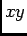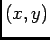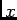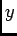# OOF: Finite Element Analysis of Microstructures

/output/cross_section/uniform/functionNext: /output/cross_section/nonuniform/function Up: /output/cross_section Previous: /output/cross_section   Contents

Subsections

## /output/cross_section/uniform/function

Plot the given function along a given line in theplane, using uniformly spaced plot points.

### cross_section/uniform arguments

filename
Where the data will go.
start
Thecoordinates of the beginning of the line along which the data will be plotted. The coordinates must be given as a comma-separated pair in parentheses, e.g, (1.2, 3.4). The coordinates are in the coordinate system of the screen, so to plot values relative to the coordinate system of the undistorted mesh, make sure to set /graphics/enhancement=0 (see sections 3.9.3 and 5.2.6).
finish
Thecoordinates of the end of the line.
npts
The number of points at which to plot the function.
x
Should thecoordinate of the plotted points be printed?
y
Should thecoordinate of the plotted points be printed?
s
Should the arclength coordinate of the plotted points be printed? The arclength is the distance from the starting point.

/* Send mail to the OOF Team *//* Go to the OOF Home Page */